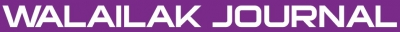### A Collocation Method for Numerical Solution of the Generalized Burgers-Huxley Equation

#### Abstract

In this paper, we use a collocation method to solve the Burgers-Huxley equation. To achieve this aim, we use mesh free technique based on sinc functions. The stability analysis is discussed. Some numerical examples are provided to illustrate the accuracy and fluency of the method.

doi:10.14456/WJST.2014.89

#### Keywords

Collocation method, Burgers-Huxley equation, Sinc function, Stability analysis

PDF

#### References

J Satsuma. Topics in Soliton Theory and Exactly Solvable Nonlinear Equations. World Scientific, Singapore, 1987, p. 255-62.

XY Wang, ZS Zhu and YK Lu. Solitary wave solutions of the generalized Burger’s-Huxley equation. J. Phys. A Math. Gen. 1990; 23, 271-4.

AL Hodgkin and AF Huxley. A quantitative description of membrane current and its application to conduction and excitation in nerve. J. Physiol. 1952; 117, 500-44.

R Fitzhugh. Mathematical Models of Excitation and Propagation in Nerve. In: HP Schwan (ed.). Biological Engineering. McGraw-Hill, New York, 1969, p. 1-85.

HNA Ismail, K Raslan and AAA Rabboh. Adomian decomposition method for Burger’s-Huxley and Burger’s Fisher equations. Appl. Math. Comput. 2004; 159, 291-301.

M Javidi. A numerical solution of the generalized Burger’s-Huxley equation by spectral collocation method, Appl. Math. Comput. 2006; 178, 338-44.

X Deng. Traveling wave solutions for the generalized Burgers-Huxley equation. Appl. Math. Comput. 2008; 204, 733-7.

F Stenger. Numerical Methods Based on Sinc and Analytic Functions. Springer-Verlag, New York, 1993.

REAC Paley and N Wiener. Fourier Transforms in the Complex Domain Colloquium Publication. vol. XIX, American Mathematical Society, 1964.

R Mokhtari and M Mohammadi. Numerical solution of GRLW equation using Sinc-collocation method. Comput. Phys. Comm. 2010; 181, 1266-74.

BVR Kumar, V Sangwan, SVSSNVGK Murthy and M Nigam. A numerical study of singularly perturbed generalized Burgers-Huxley equation using three-step Taylor-Galerkin method. Comput. Math. Appl. 2011; 62, 776-86.

JE Macias-Diaz, J Ruiz-Ramirez and J Villa. The numerical solution of a generalized Burgers-Huxley equation through a conditionally bounded and symmetry-preserving method. Comput. Math. Appl. 2011; 61, 3330-42.

M Javidi and A Golbabai. A new domain decomposition algorithm for generalized Burger’s-Huxley equation based on Chebyshev polynomials and preconditioning. Chaos Soliton. Fract. 2009; 39, 849-57.

A Molabahrami and F Khani. The homotopy analysis method to solve the Burgers-Huxley equation. Nonlinear Anal. R. World Appl. 2009; 10, 589-600.

M Sari and G Gurarslan. Numerical solutions of the generalized Burgers-Huxley equation by a differential quadrature method. Math. Probl. Eng. 2009; 2009, Article ID 370765.

OY Efimova and NA Kudryashov. Exact solutions of the Burgers-Huxley equation. J. Appl. Math. Mech. 2004; 60, 413-20.

NK Vitanove. Application of simplest equation of Bernoulli and Riccatti kind for obtaining exact traveling-wave solutions for a class of PDEs with polynomial nonlinearity. Comm. Nonlinear Sci. Numer. Simulat. 2010; 15, 2050-60.

### Refbacks

• There are currently no refbacks.Online ISSN: 2228-835X

http://wjst.wu.ac.th

Last updated: 20 June 2019